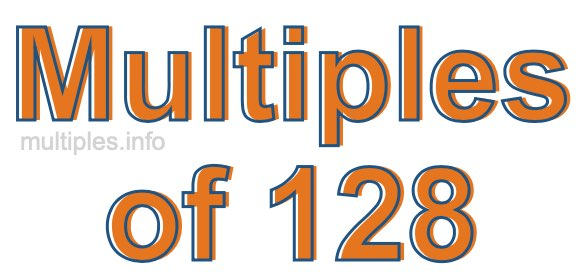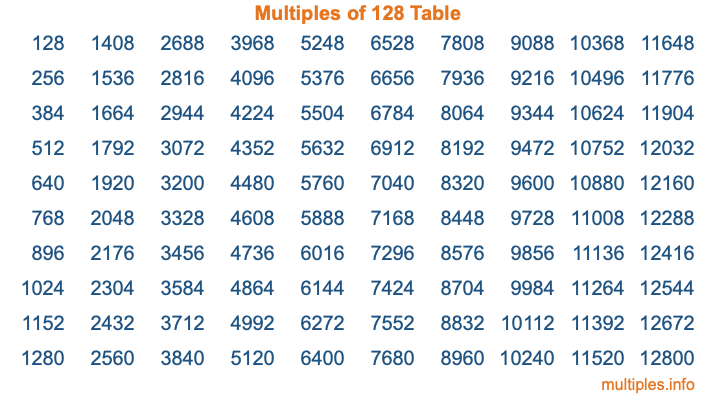Multiples of 128Welcome to the Multiples of 128 page. Here we will first teach you everything you will ever need to know about the multiples of 128, and then give you a study guide summary of everything we taught you to make sure you remember it all. Use this page to look up facts and learn information about the multiples of 128. This page will make you a multiples of one hundred twenty-eight expert!

Definition of Multiples of 128
Multiples of 128 are all the numbers that when divided by 128 equal an integer. Each of the multiples of 128 are called a multiple. A multiple of 128 is created by multiplying 128 by an integer.

Therefore, to create a list of multiples of 128, you start with 1 multiplied by 128, then 2 multiplied by 128, then 3 multiplied by 128, and so on for as long as you want. Thus, the list of the first five multiples of 128 is 128, 256, 384, 512, and 640. To see a larger list of multiples of 128, see the printable image of Multiples of 128 further down on this page. We also have a category where you can choose any nth multiple of 128.

Multiples of 128 Checker
The Multiples of 128 Checker below checks to see if any number of your choice is a multiple of 128. In other words, it checks to see if there is any number (integer) that when multiplied by 128 will equal your number. To do that, we divide your number by 128. If the the quotient is an integer, then your number is a multiple of 128.

Is  a multiple of 128?

Least Common Multiple of 128 and ...
A Least Common Multiple (LCM) is the lowest multiple that two or more numbers have in common. This is also called the smallest common multiple or lowest common multiple and is useful to know when you are adding our subtracting fractions. Enter one or more numbers below (128 is already entered) to find the LCM.

Check out our LCM Calculator if you need more details about the Least Common Multiple or if you need the LCM for different numbers for adding and subtraction fractions.

nth Multiple of 128
As we stated above, 128 is the first multiple of 128, 256 is the second multiple of 128, 384 is the third multiple of 128, and so on. Enter a number below to find the nth multiple of 128.

th multiple of 128

Multiples of 128 vs Factors of 128
128 is a multiple of 128 and a factor of 128, but that is where the similarities end. All postive multiples of 128 are 128 or greater than 128. All positive factors of 128 are 128 or less than 128.

Below is the beginning list of multiples of 128 and the factors of 128 so you can compare:

Multiples of 128: 128, 256, 384, 512, 640, etc.

Factors of 128: 1, 2, 4, 8, 16, 32, 64, 128

As you can see, the multiples of 128 are all the numbers that you can divide by 128 to get a whole number. The factors of 128, on the other hand, are all the whole numbers that you can multiply by another whole number to get 128.

It's also interesting to note that if a number (x) is a factor of 128, then 128 will also be a multiple of that number (x).

Multiples of 128 vs Divisors of 128
The divisors of 128 are all the integers that 128 can be divided by evenly. Below is a list of the divisors of 128.

Divisors of 128: 1, 2, 4, 8, 16, 32, 64, 128

The interesting thing to note here is that if you take any multiple of 128 and divide it by a divisor of 128, you will see that the quotient is an integer.

Multiples of 128 Table
Below is an image of the first 100 multiples of 128 in a table. The table is in chronological order, column by column. The first column has the first ten multiples of 128, the second column has the next ten multiples of 128, and so on.The Multiples of 128 Table is also referred to as the 128 Times Table or Times Table of 128. You are welcome to print out our table for your studies.

Negative Multiples of 128
Although not often discussed or needed in math, it is worth mentioning that you can make a list of negative multiples of 128 by multiplying 128 by -1, then by -2, then by -3, and so on, to get the following list of negative multiples of 128:

-128, -256, -384, -512, -640, etc.

Multiples of 128 Summary
Below is a summary of important Multiples of 128 facts that we have discussed on this page. To retain the knowledge on this page, we recommend that you read through the summary and explain to yourself or a study partner why they hold true.

There are an infinite number of multiples of 128.

A multiple of 128 divided by 128 will equal a whole number.

128 divided by a factor of 128 equals a divisor of 128.

The nth multiple of 128 is n times 128.

The largest factor of 128 is equal to the first positive multiple of 128.

128 is a multiple of every factor of 128.

128 is a multiple of 128.

A multiple of 128 divided by a divisor of 128 equals an integer.

128 divided by a divisor of 128 equals a factor of 128.

Any integer times 128 will equal a multiple of 128.

Multiples of a Number
Here you can get the multiples of another number, all with the same attention to detail as we did for multiples of 128 on this page.

Multiples of
Multiples of 129
Did you find our page about multiples of one hundred twenty-eight educational? Do you want more knowledge? Check out the multiples of the next number on our list!

Copyright  |   Privacy Policy  |   Disclaimer  |   Contact